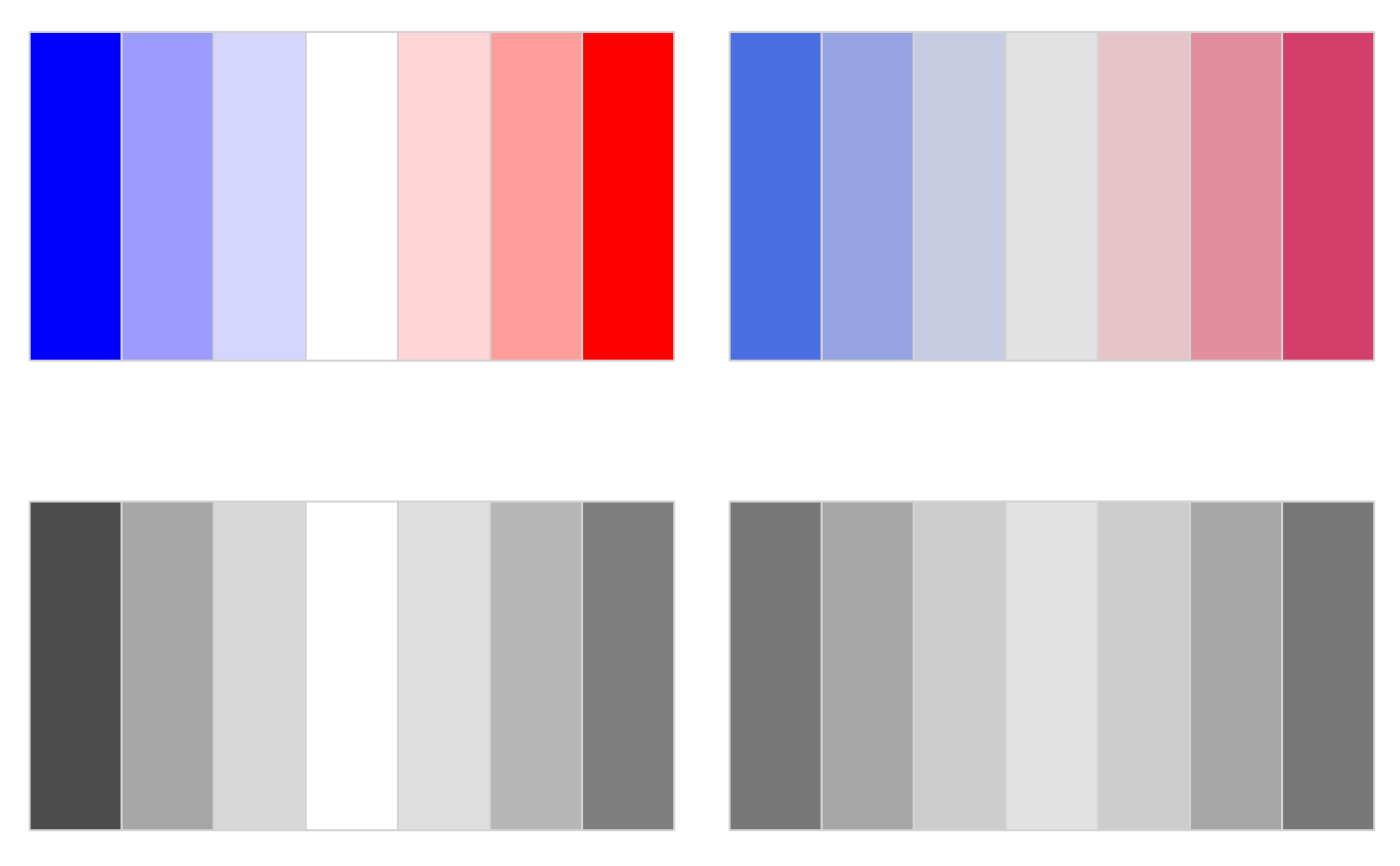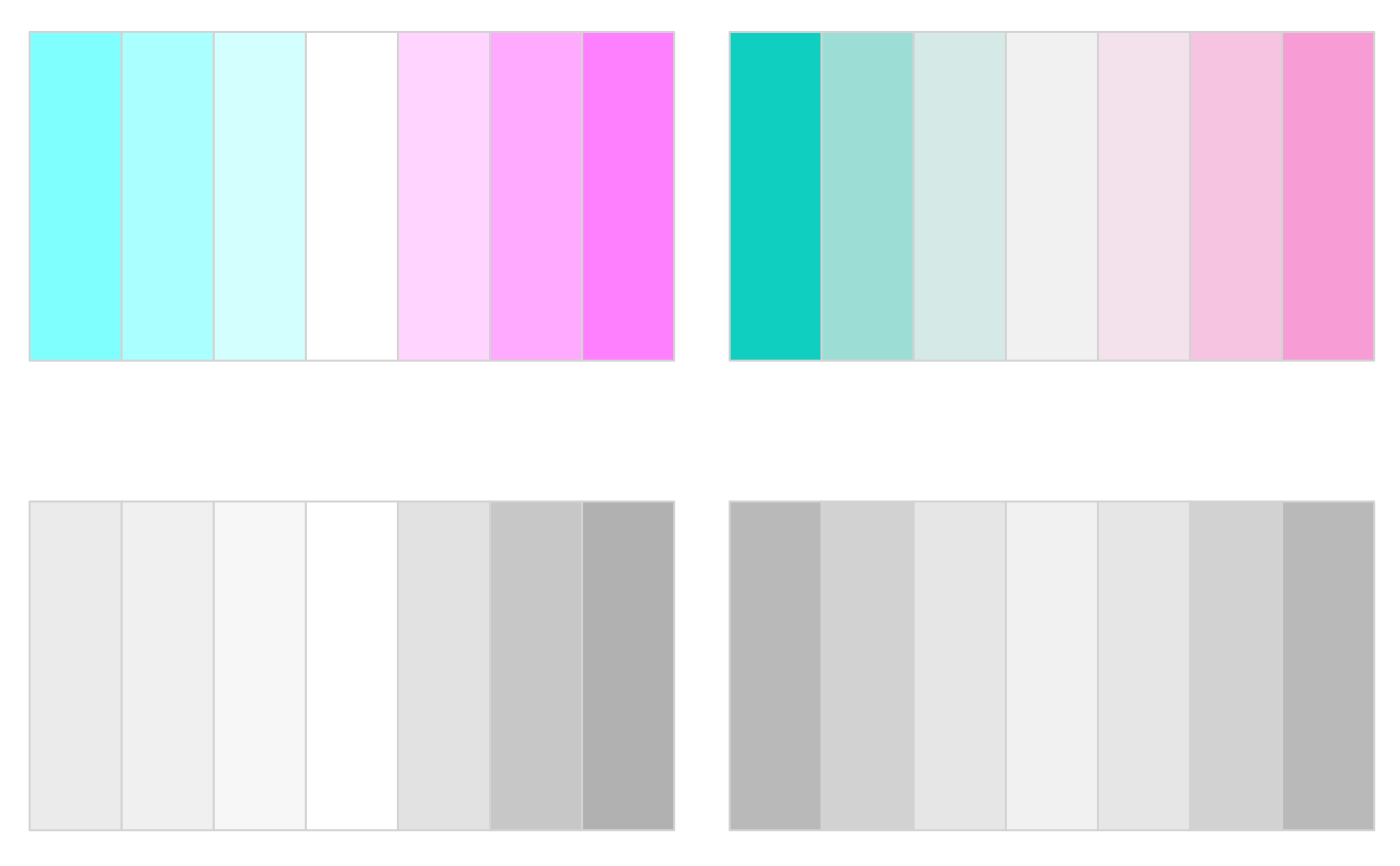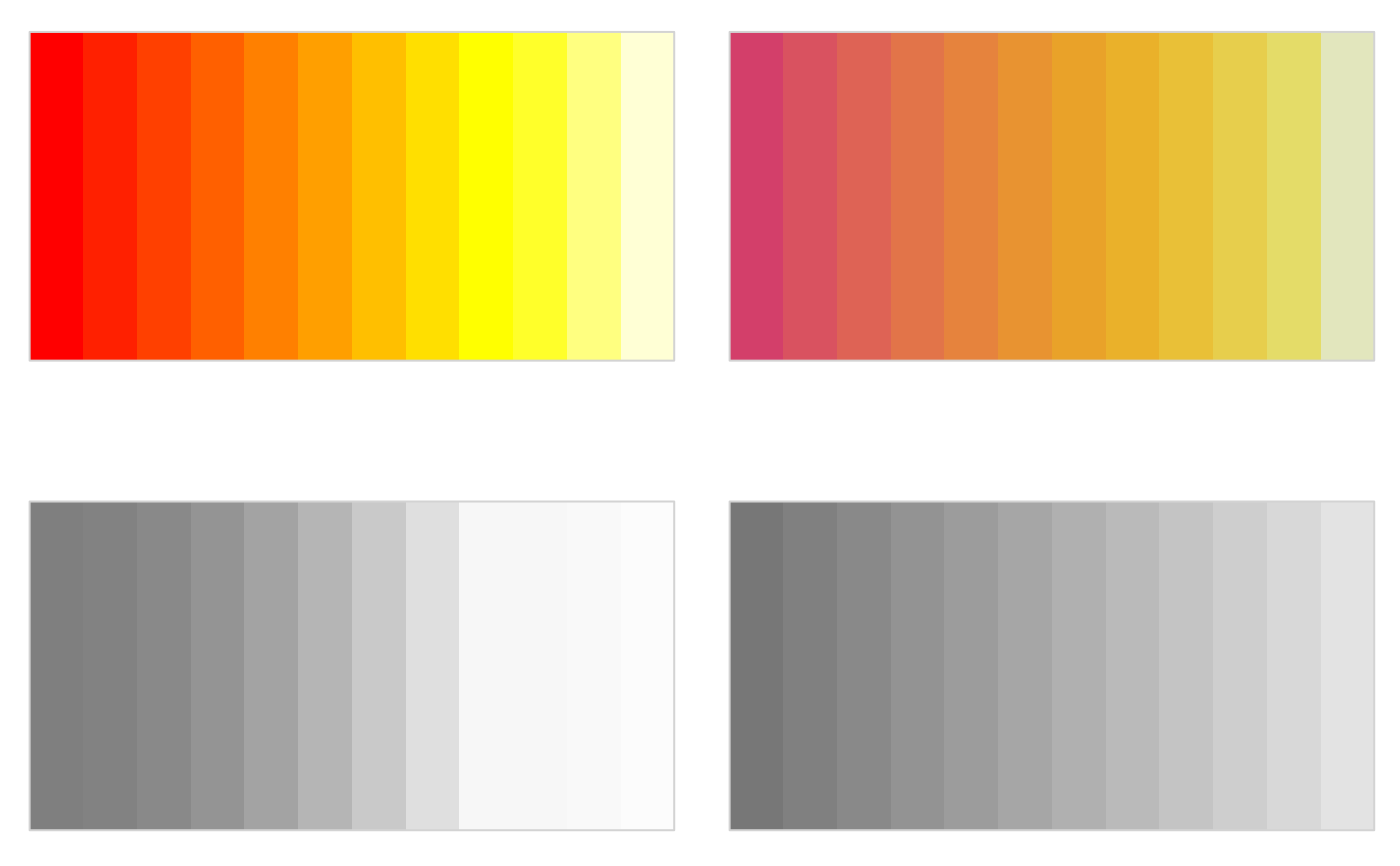Color palettes based on the HCL (and HSV) color space to replace base R palettes.

rainbow_hcl(
n,
c = 50,
l = 70,
start = 0,
end = 360 * (n - 1)/n,
gamma = NULL,
fixup = TRUE,
alpha = 1,
...
)

heat_hcl(
n,
h = c(0, 90),
c. = c(100, 30),
l = c(50, 90),
power = c(1/5, 1),
gamma = NULL,
fixup = TRUE,
alpha = 1,
...
)

terrain_hcl(
n,
h = c(130, 0),
c. = c(80, 0),
l = c(60, 95),
power = c(1/10, 1),
gamma = NULL,
fixup = TRUE,
alpha = 1,
...
)

diverging_hsv(
n,
h = c(240, 0),
s = 1,
v = 1,
power = 1,
gamma = NULL,
fixup = TRUE,
alpha = 1,
...
)

## Arguments

n

the number of colors ($$\ge 1$$) to be in the palette.

c, c.

chroma value in the HCL color description.

l

luminance value in the HCL color description.

start

the hue at which the rainbow begins.

end

the hue at which the rainbow ends.

gamma

Deprecated.

fixup

logical. Should the color be corrected to a valid RGB value before correction?

alpha

numeric vector of values in the range [0, 1] for alpha transparency channel (0 means transparent and 1 means opaque).

...

Other arguments passed to hex.

h

hue value in the HCL or HSV color description, has to be in [0, 360] for HCL and in [0, 1] for HSV colors.

power

control parameter determining how chroma and luminance should be increased (1 = linear, 2 = quadratic, etc.).

s

saturation value in the HSV color description.

v

value value in the HSV color description.

## Value

A character vector with (s)RGB codings of the colors in the palette.

## Details

Based on the general qualitative, sequential, and diverging hcl_palettes within the colorspace package, convenience functions are provided as alternatives to standard base R palettes (which are highly saturated and too flashy).

rainbow_hcl computes a rainbow of colors via qualitative_hcl defined by different hues given a single value of each chroma and luminance. It corresponds to rainbow which computes a rainbow in HSV space.

heat_hcl is an implementation of heat.colors in HCL space based on a call to sequential_hcl. Similarly, terrain_hcl palette also calls sequential_hcl with different parameters, providing colors similar in spirit to terrain.colors in HCL space.

diverging_hsv (and equivalently its alias diverge_hsv) provides an HSV-based version of diverging_hcl. Its purpose is mainly didactic to show that HSV-based diverging palettes are less appealing, more difficult to read and more flashy than HCL-based diverging palettes. diverging_hsv is similar to cm.colors.

polarLUV, HSV, hex

## Examples

## convenience demo function
wheel <- function(col, radius = 1, ...)

## compare base and colorspace palettes
## (in color and desaturated)
par(mar = rep(0, 4), mfrow = c(2, 2))
## rainbow color wheel
wheel(rainbow_hcl(12))
wheel(rainbow(12))
wheel(desaturate(rainbow_hcl(12)))
wheel(desaturate(rainbow(12)))## diverging red-blue colors
swatchplot(
diverging_hsv(7),
desaturate(diverging_hsv(7)),
diverging_hcl(7, c = 100, l = c(50, 90)),
desaturate(diverging_hcl(7, c = 100, l = c(50, 90))),
nrow = 2
)## diverging cyan-magenta colors
swatchplot(
cm.colors(7),
desaturate(cm.colors(7)),
diverging_hcl(7, "Cyan-Magenta"), ## or, similarly: Tropic
desaturate(diverging_hcl(7, "Cyan-Magenta")),
nrow = 2
)## heat colors
swatchplot(
heat.colors(12),
desaturate(heat.colors(12)),
heat_hcl(12),
desaturate(heat_hcl(12)),
nrow = 2
)## terrain colors
swatchplot(
terrain.colors(12),
desaturate(terrain.colors(12)),
terrain_hcl(12),
desaturate(terrain_hcl(12)),
nrow = 2
)